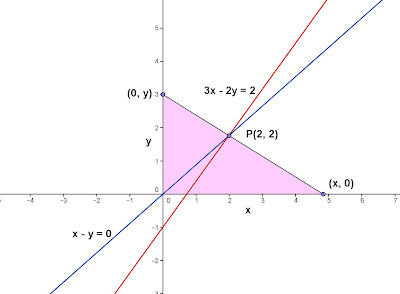## Tuesday, July 23, 2013

### Two Intersecting Lines, 3

Category: Analytic Geometry, Algebra

"Published in Newark, California, USA"

Find the equation of a line that passes through the intersection of x - y = 0 and 3x - 2y = 2 and forms a triangle at 1st Quadrant whose area is 9.

Solution:

To illustrate the problem, it is better to sketch the graph of two lines as follows:

For x - y = 0,

x - y = 0
y = x

slope (∆y/∆x), m = 1
y-intercept = 0

To trace the graph, plot 0 at the y-axis. This is your first point of the line (0, 0). Next, use the slope to get the second point. From the first point, count 1 unit to the right and then 1 unit upward.

For 3x - 2y = 2,

3x - 2y = 2
2y = 3x - 2
y = 3/2 x - 1

slope (∆y/∆x), m = 3/2
y-intercept = -1

To trace the graph, plot -1 at the y-axis. This is your first point of the line (0, -1). Next, use the slope to get the second point. From the first point, count 2 units to the right and then 3 units upward.Photo by Math Principles in Everyday Life

To get their point of intersection, we have to use the two given equations and solve for x and y as follows

from

Substitute the second equation to the first equation, we have

Substitute x to either of the two equations,

Therefore, their point of intersection is P(2, 2).Photo by Math Principles in Everyday Life

From the given word problem says "...forms a triangle at 1st Quadrant whose area is 9.", then the line that passes through point P will intersect at x-axis and y-axis. The resulting figure is a right triangle whose sides are x and y. The right angle is the origin while point P is located at the hypotenuse as followsPhoto by Math Principles in Everyday Life

By using the formula for the area of a triangle, the first equation will be

By using the formula for getting the slope of two points, the second equation will be

Substitute the value of y from the first equation to the second equation, we have

Multiply both sides of the equation by x

If you will choose the positive sign,

Substitute the value of x either to the first equation or second equation, we have

The slope of a line is calculated as follows

Therefore, using the Point-Slope Form, the equation of a line is

If you will choose the negative sign,

Substitute the value of x either to the first equation or second equation, we have

The slope of a line is calculated as follows

Therefore, using the Point-Slope Form, the equation of a line is